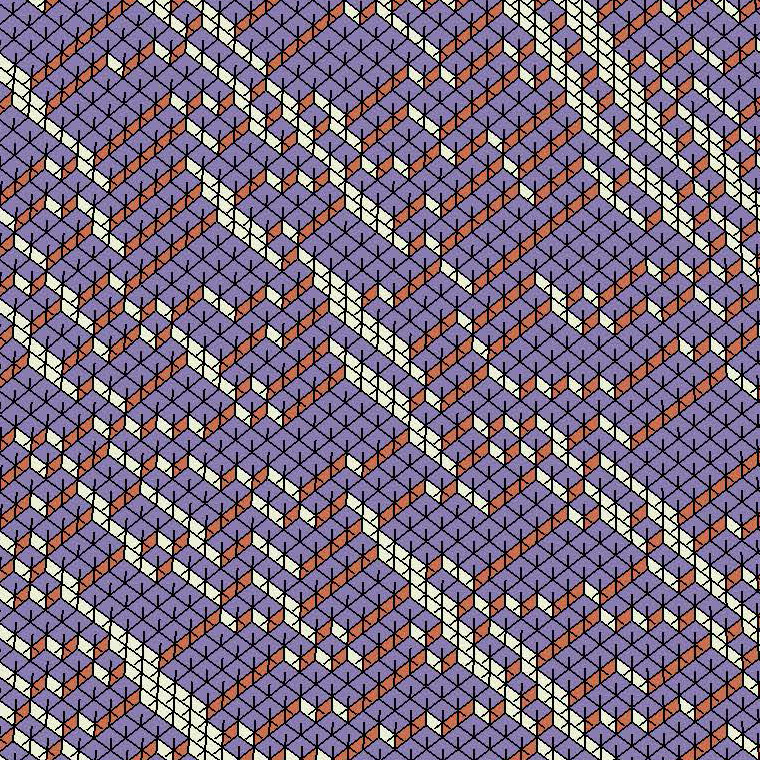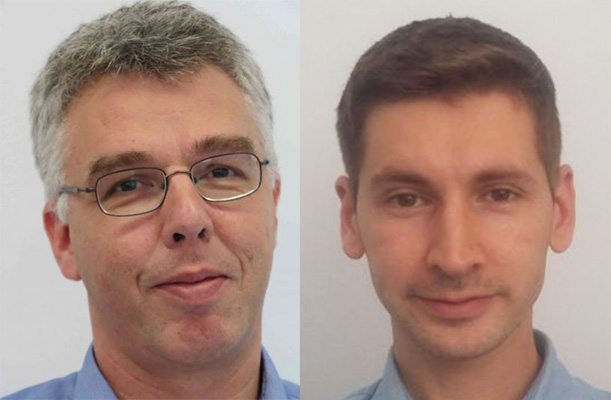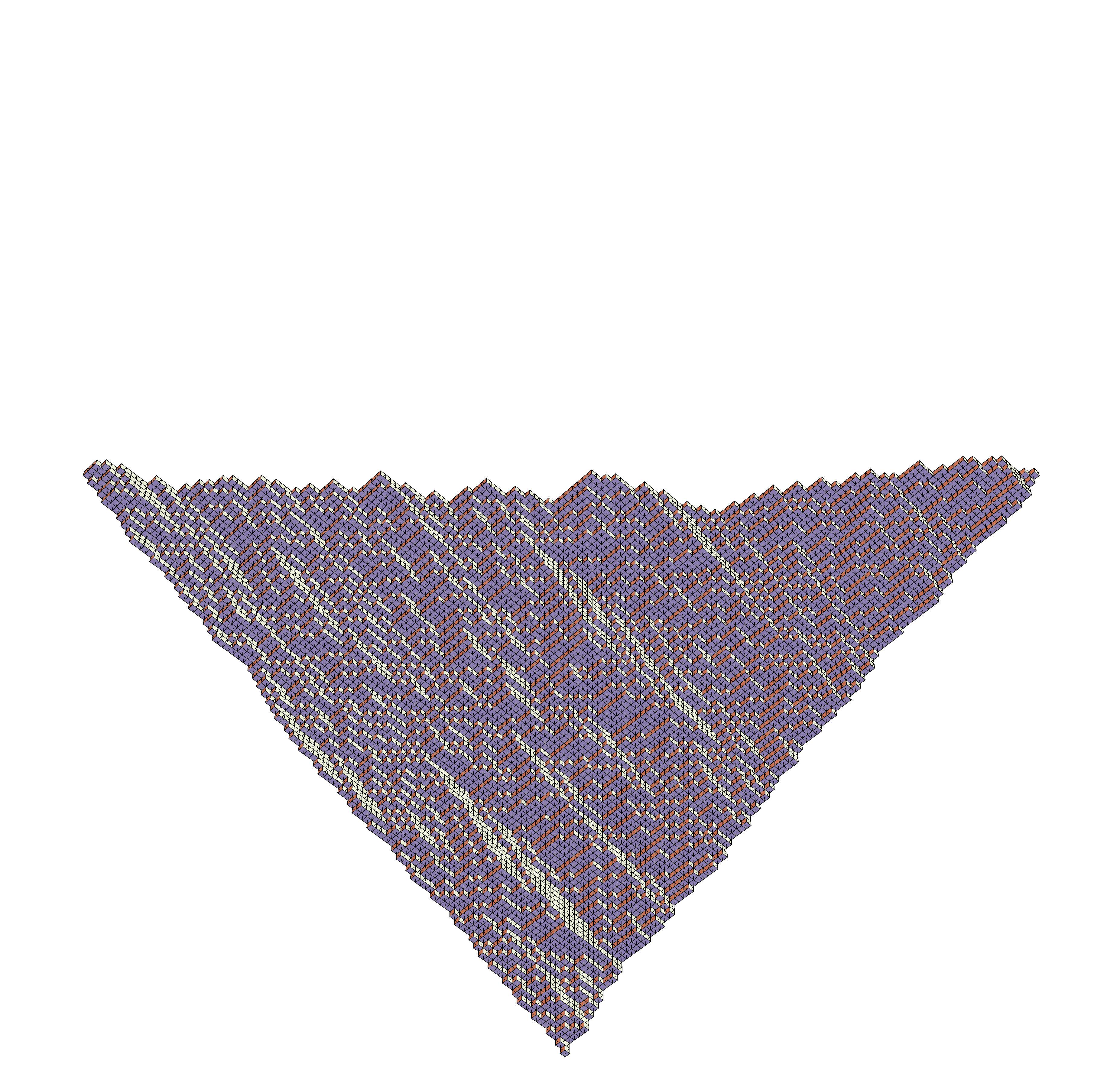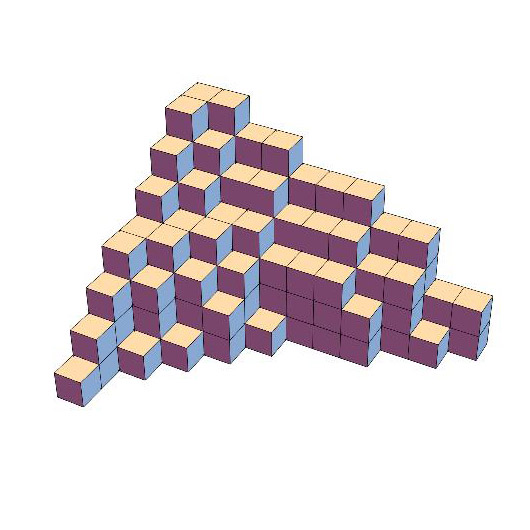# Case Study: Constructing mathematical models from quantum toroidal algebrasDetail of a plane partition representing a surface of a crystal or a fluctuating membrane

ACEMS researchers use abstract symmetries, called Lie algebras, to derive universal predictions from mathematical models that describe randomness in nature, for example in interface growth. At The University of Melbourne, researchers Professor Jan de Gier and Dr Alexandr (Sasha) Garbali, have just completed research which contributes to quantum versions of toroidal Lie algebras, called quantum toroidal algebras.

Rational functions in two variables have the x-y plane as their natural domain. For trigonometric, or periodic, functions we can think of a cylinder as the natural domain since the values of the functions are repeated as we trace paths around the cylinder. This hierarchy of functions is topped by doubly periodic elliptic functions which are naturally defined over the torus.

During the last century, the same trichotomy from plane to cylinder to torus has been found in integrable systems, symmetry algebras and more abstract mathematical structures such as cohomology groups. In particular, the relationship applies between Lie algebras, affine Lie algebras and toroidal Lie algebras. And, like the theory of functions, the relationship among integrable systems, algebra, geometry and physics becomes most interesting at the elliptic level.

“Quantum toroidal algebras are at the forefront of modern mathematical research and a hot topic internationally. We started reading up on literature in 2016 and thought we could contribute given our skills and background,” says Jan, a Chief Investigator with ACEMS.

From a mathematical perspective, Jan says that these algebras incorporate and generalise many known abstract symmetry algebras. It is a new and intriguing challenge to unravel and understand their structure in a precise way.

“They also seem to pop-up as underlying unifying structures in several modern physics developments, including in string theory, supersymmetric gauge theories, condensed matter systems and interacting particle models,” says Jan.

Quantised affine Lie algebras, for example, underpin the integrability of (multi-species) asymmetric exclusion processes. The existence of these powerful underlying symmetry algebras ultimately leads to the rigorous derivation of universal distribution functions for particle currents and similar observables that hold for a very broad class of stochastic systems and are related to the theory of random matrices.

On the other hand, quantum toroidal algebras can be considered as a parent theory, standing at the top of the symmetry hierarchy. Other algebras can be considered as simpler cases. Much of the representation theory of quantum toroidal algebras is yet to be discovered, as are its consequences for applications to physical systems.

Jan says they expect important conclusions for two-dimensional stochastic systems that generalise the one-dimensional exclusion processes.

“Because plane partitions (see figure) play such an important role in the mathematical analysis of toroidal algebras, we are exploring how we can apply quantum toroidal algebras in new and different ways, for example to the design of two-dimensional stochastic models of membrane growth,” says Jan.

To unravel this, Jan says he and Sasha will need to construct the so-called R-matrix to provide explicit expressions for stochastic generators and Hamiltonians in physics applications. The problem of constructing the R-matrix is rather complicated and mathematically advanced.

“Our paper makes significant progress in developing a method for its explicit construction in the case of the most fundamental quantum toroidal algebras. This method can likely be generalised to other quantum toroidal algebras,” says Jan.

Jan says this type of mathematical research takes time, but it is vital that it gets done. He likens it to someone trying to find their way through a dense forest where they have not been before.

“Once you have made your way through, you can probably retrace your steps and then walk it a second time, but now it is a bit easier because you know where you are going. Then over time as the steps get tracked by others, it becomes a trail and eventually a well-trodden path, extending the known world of mathematics,” says Jan.

Thanks to Jan and Sasha, there is a path now for others to follow. As of this writing, their research has been accepted into the top journal in their field, Communications in Mathematical Physics.

### The PeopleProfessor Jan de Gier and Dr Alexandr (Sasha) GarbaliA plane partition representing a surface of a crystal or a fluctuating membraneA plane partition representing a surface of a crystal or a fluctuating membrane